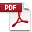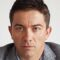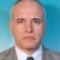# BRUTOMIZER 1.1

You no longer need an expert for multi-objective optimization!

START OPTIMIZING WITH BRUTOMIZER!

Search through ALL possible input values to obtain solution(s) that are GUARANTEED to be optimal.

Ideal for solving real engineering optimization problems, where inputs take values from discrete space, and are not continual.

BRUTOMIZER Ver 1.1 FREE

Supported OSs: MS Windows XP / Vista / 7 / 8 / 10 / 11

Prerequisite: Microsoft .NET Framework 4.5BRUTOMIZER Manual

BRUTOMIZER provides two essential features:

Find min/max – find optimal value(s) of output function (and corresponding inputs)

Target value – find specific output function value, with defined accuracy (and corresponding inputs)

When BRUTOMIZER?

When you solve real engineering problems (where input parameters take values from discrete set of known values).

When you validate some newly developed heuristic optimization method.

Why BRUTOMIZER?

Because no expert knowledge is needed.

Because optimality of provided solution(s) is guaranteed.

Because it is fast, reliable and easy to use.

Key features:

• Define start value, end value and step for each input variable
• Define one or more mathematical models (i.e. output functions)
• Define zero or more constraints
• Select working mathematical model (which will be optimized)
• Select zero or more working constraints (which will be considered during optimization)
• Select zero or more watched mathematical models (which will be observed during optimization)
• Find one or more minimums (maximums) of selected working mathematical model (considering selected working constraints)
• Target specific value of working mathematical model (considering selected working constraints), with defined accuracy
• Save load all set parameters to/from project file

Limitations:

• Up to 8 input variables
• Up to  99 999 999 999 input values combinations
• Up to 100 maximums (minimums) in one optimization run

OPTIMALITY GUARANTEED!

BRUTOMIZER is based on brute force iterative search of ALL possible input values. This approach GUARANTEES that obtained solution(s) are OPTIMAL in defined discrete search space. Further more, corresponding inputs can be readily set as parameters of the process being optimized.

OPTIMALITY GUARANTEED!

BRUTOMIZER is based on brute force iterative search of ALL possible input values. This approach GUARANTEES that obtained solution(s) are OPTIMAL in defined discrete search space. Further more, corresponding inputs can be readily set as parameters of the process being optimized.

SPEED!

By utilizing multiple processor cores and smart memory management, billions of input combinations can be handled in a matter of minutes.

EASILY DEFINE ANY MATHEMATICAL MODEL!

Definition of any mathematical model is available through the “General” model option (powered by NCalc) from the BRUTOMIZER user interface.

However, vast majority of real engineering problems are empirically modeled using regression analysis by means of first and second order polynomials, with 2-way interactions. If dealing with such mathematical models and calculation speed is important, then it is better to use “Regression model of order 2” option from the BRUTOMIZER user interface.

CUSTOMIZED MODELS FOR INCREASED PERFORMANCE!

If You are dealing with billions of input combinations and complex mathematical models, it is smart to have Your mathematical models custom developed – it can improve performance up to 100 times.

Custom mathematical models can become available for selection in BRUTOMIZER, just for You! For a reasonable price You can save hours of work! Contact us!

Case Studies:

1. Pressure vessel design case study

2. Linear programming – Product-mix case study